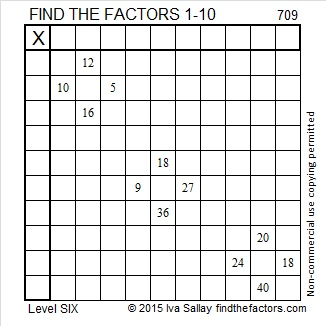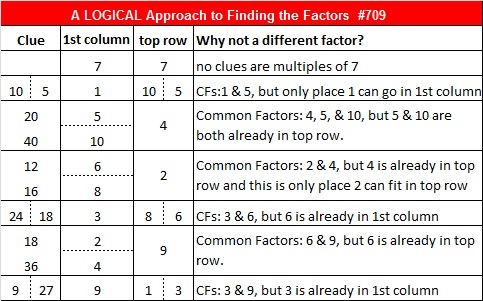# 709 and Level 6

• 709 is a prime number.
• Prime factorization: 709 is prime.
• The exponent of prime number 709 is 1. Adding 1 to that exponent we get (1 + 1) = 2. Therefore 709 has exactly 2 factors.
• Factors of 709: 1, 709
• Factor pairs: 709 = 1 x 709
• 709 has no square factors that allow its square root to be simplified. √709 ≈ 26.62705.How do we know that 709 is a prime number? If 709 were not a prime number, then it would be divisible by at least one prime number less than or equal to √709 ≈ 26.6. Since 709 cannot be divided evenly by 2, 3, 5, 7, 11, 13, 17, 19, or 23, we know that 709 is a prime number.

Here is today’s puzzle:Print the puzzles or type the solution on this excel file: 10 Factors 2015-12-07

———————————————————————————

Here’s another way we know that 709 is a prime number: Since 22² + 15² = 709, and 22 and 15 have no common prime factors, 709 will be prime unless it is divisible by a primitive Pythagorean hypotenuse less than or equal to √709 ≈ 26.6. Since 709 is not divisible by 5, 13, or 17, we know that 709 is a prime number.

354 + 355 = 709. That is the only way 709 is the sum of consecutive counting numbers.

Because 22² + 15² = 709, it is the hypotenuse of the primitive Pythagorean triple 259-660-709 which was calculated using 22² – 15², 2(22)(15), 22² + 15².

709 is palindrome 595 in BASE 11; note that 5(121) + 9(11) + 5(1) = 709.

———————————————————————————Ricardo has tweeted the solution, too: